Welcome to E-Club40

# Introducing our 2x14 Forced Matrix System

Introducing our 2x14 Forced Matrix System
Requirement: 2 Direct Referrals
Regular Membership (P40) - Earn P31,611 Net Income for each account + BenefitsTerminologies:

2x14: 2 wide, 14 level deep. Every single member can pay to you.
Forced: means that you cannot place your new people where you want them; they must fall in the next open space, typically filled from top to bottom.
Matrix System: based around a compensation structure that has a set width and depth for which members are compensate
D.R or Direct Referrals: are people that you directly refer to E-Club
I.R or Indirect Referrals: are people that are referred by your direct referrals

How Matrix Works?
Matrix get filled 4 WAYS BY TEAM EFFORT:

Filling a Matrix will not only come from your own effort. It may come from your upline, downline, crossline and even from other members that do not have a position here in our 2x14 Forced Matrix

1. Direct Referrals – Your referrals will always follow you or be place on the next available position on your current matrix

2. Spill over – spill over may come from the referrals of the people above you  of other members from our progressing cycling matrix

3. Spill under -  this are the referrals under your matrix

4. Re-entry position – this are new position
E Club Compensation Plan DetailsRegular Membership (P40)

Section 1= 2 D.R (Earn P2 each) = P4
Section 2= 4 I.R (Earn P2 each) = P8
Section 3= 8 I.R (Earn P2 each) = P16
Section 4= 16 I.R (Earn P2each) = P32
Section 5= 32 I.R (Earn P2 each) = P64
Section 6= 64 I.R (Earn P2 each) = P128
Section 7= 128 I.R (Earn P2 each) = P256
Section 8= 256 I.R (Earn P2 each) = P512
Section 9= 512 I.R (Earn P2 each) = P1024
Section 10= 1024 I.R (Earn P2 each) = P2048
Section 11= 2048 I.R (Earn P2 each) = P4096
Section 12= 4096 I.R (Earn P2 each) = P8192
Section 13= 8192 I.R (Earn P2 each) = P16384
Section l14= 16384 I.R (Earn P2 each) = P32768
Total of P65,532 GROSS INCOMENote: P10,000 - Maximum withdrawal if Multiple Accounts to avoid over payout (weekly).

LUCKY 6,7,8 Bonus: Once you reached Section 6 with 64 Indirect Referrals you will get a FREE Worldwide Job and Immigration Opportunities Seminar (save P500). If you reached in Section 7 (128 Indirect Referrals), get a P45,000 worth of Accident Insurance. Lastly, if you reached section 8 with 256 Indirect Referrals you will get a P500 SM gift certificate.
Premium Membership (P400)

Level 1, Day 1 = 2 D.R (Earn P20each) =P40
Level 2, Day 2 = 4 I.R (Earn P20each) = P80
Level 3, Day 3 =8 I.R (Earn P20each) = P160
Level 4, Day 4 = 16 I.R (Earn P20each) = P320
Level 5, Day 5 = 32 I.R (Earn P20each) = P640
Level 6, Day 6 = 64 I.R (Earn P20each) = P1280
Level 7, Day 7 = 128 I.R (Earn P20each) = P2560
Level 8, Day 8 = 256 I.R (Earn P20each) =P5120
Level 9, Day 9 = 512 I.R (Earn P20each) = P10240
Level 10, Day 10 = 1024 I.R (Earn P20each) =P20480
Level 11, Day11 = 2048 I.R (Earn P20each) =P40960
Level 12, Day 12=  4096 I.R (Earn P20each) = P81920
Level 13, Day 13 = 8192 I.R (Earn P20each) =P163,840
Level 14, Day 14 =16384 I.R(Earn P20each) = P327,680

Total Gross Income = P655,320Note: P50,000 - Maximum withdrawal if Multiple Accounts to avoid over payout (weekly).

Cash Out Details

Request of Commission: Saturday and Sunday = From 10:AM-10PM

Processing Days: Monday-Thursday

Pay Day: Friday

Payment Transaction
• Personal bank account or GCASH,
• Door2Door Cash Delivery
• Nearest Remittances in your area.
WHAT DO YOU GET FROM YOUR MEMBERSHIP FEE?
Regular Membership (P40)
o Quality Ebooks (choose 40 ebooks from the 55,000 ebooks available)
o Personal Back Office Account
o Enrolled in our E Study Center (will soon expand nationwide)
o Free 2 hrs use of E-Study center (study,internet,unwind Davao Branch Only)
o Free Online Career Assessment (save P500)
o Discount to all our E-Services

E-CLUB FINANCIAL LOAN FOR STUDENTS AND MEMBERS
GUIDELINES:
NO COLLATERAL
COMMISSION DEDUCTION BASIS
1st to Reserve 1st to Cater Basis
5-7 Days Processing
Payment Terms: 3 Consecutive Payouts
5% Interest

Regular Members (P40)
Requirement: E-Club members should have the 2 Direct Referrals and the following number of Indirect Referrals to avail our student loan assistance.
128 Indirect Referrals = P500
256 Indirect Referrals = P1,000
512 Indirect Referrals = P2,000
1024 Indirect Referrals = P3,000
2048 Indirect Referrals = P4,000
4096 Indirect Referrals = P10,000

Comments on this page:
 Comment posted by ecar( maricar_cuaresma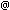yahoo.com ), 01/11/2015 at 11:34am (UTC):maam, sir may pay-out po aq n 900 pesos since 2014 pero bakit po d q n maopen ung account q sa eclub

Add comment to this page:
 Your Name: Your Email address: Your message: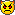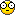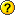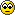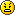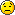© 2013 E-Club40. All Rights Reserved.